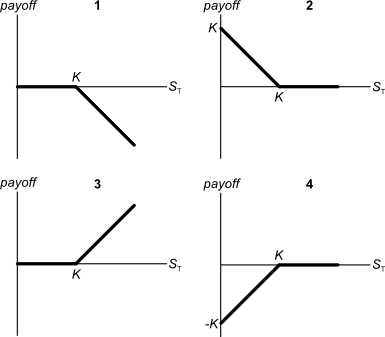# Fight Finance

#### CoursesTagsRandomAllRecentScores

If a project's net present value (NPV) is zero, then its internal rate of return (IRR) will be:

Below are 4 option graphs. Note that the y-axis is payoff at maturity (T). What options do they depict? List them in the order that they are numberedYour neighbour asks you for a loan of $100 and offers to pay you back$120 in one year.

You don't actually have any money right now, but you can borrow and lend from the bank at a rate of 10% pa. Rates are given as effective annual rates.

Assume that your neighbour will definitely pay you back. Ignore interest tax shields and transaction costs.

The Net Present Value (NPV) of lending to your neighbour is $9.09. Describe what you would do to actually receive a$9.09 cash flow right now with zero net cash flows in the future.

Find Scubar Corporation's Cash Flow From Assets (CFFA), also known as Free Cash Flow to the Firm (FCFF), over the year ending 30th June 2013.

 Scubar Corp Income Statement for year ending 30th June 2013 $m Sales 200 COGS 60 Depreciation 20 Rent expense 11 Interest expense 19 Taxable Income 90 Taxes at 30% 27 Net income 63  Scubar Corp Balance Sheet as at 30th June 2013 2012$m $m Inventory 60 50 Trade debtors 19 6 Rent paid in advance 3 2 PPE 420 400 Total assets 502 458 Trade creditors 10 8 Bond liabilities 200 190 Contributed equity 130 130 Retained profits 162 130 Total L and OE 502 458 Note: All figures are given in millions of dollars ($m).

The cash flow from assets was:

A levered firm has a market value of assets of $10m. Its debt is all comprised of zero-coupon bonds which mature in one year and have a combined face value of$9.9m.

Investors are risk-neutral and therefore all debt and equity holders demand the same required return of 10% pa.

Therefore the current market capitalisation of debt $(D_0)$ is $9m and equity $(E_0)$ is$1m.

A new project presents itself which requires an investment of $2m and will provide a: •$6.6m cash flow with probability 0.5 in the good state of the world, and a
• -$4.4m (notice the negative sign) cash flow with probability 0.5 in the bad state of the world. The project can be funded using the company's excess cash, no debt or equity raisings are required. What would be the new market capitalisation of equity $(E_\text{0, with project})$ if shareholders vote to proceed with the project, and therefore should shareholders proceed with the project? The below screenshot of Commonwealth Bank of Australia's (CBA) details were taken from the Google Finance website on 7 Nov 2014. Some information has been deliberately blanked out.What was CBA's approximate payout ratio over the 2014 financial year? Note that the firm's interim and final dividends were$1.83 and $2.18 respectively over the 2014 financial year. An equity index is currently at 5,200 points. The 6 month futures price is 5,300 points and the total required return is 6% pa with continuous compounding. Each index point is worth$25.

What is the implied dividend yield as a continuously compounded rate per annum?

Here is a table of stock prices and returns. Which of the statements below the table is NOT correct?

 Price and Return Population Statistics Time Prices LGDR GDR NDR 0 100 1 50 -0.6931 0.5 -0.5 2 100 0.6931 2 1 Arithmetic average 0 1.25 0.25 Arithmetic standard deviation -0.6931 0.75 0.75

The following steps set out the process of ‘negative gearing’ an investment property in Australia. Which of these steps or statements is NOT correct? To successfully achieve negative gearing on an investment property:

Question 984  principal agent problem, moral hazard, asymmetric information, no explanation

When does the ‘principal-agent problem’ occur? Is it when:

I. The principal has conflicting incentives (moral hazard);

II. The agent has conflicting incentives (moral hazard);

III. The principal has incomplete information about the agent (asymmetric information); or

IV. The agent has incomplete information about the principal (asymmetric information)?

The principal-agent problem occurs when statements: